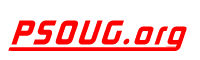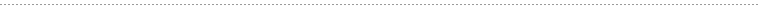Quick Search:PHP Code: Calculate date of Easter Sunday Jump to: Select a Location PSOUG Home Page Oracle Code Library mySQL Code Library PHP Code Library JavaScript Code Library Oracle Terms & Definitions Oracle Error Codes Advanced Code Search Contact UsSnippet Name: Calculate date of Easter Sunday

Description: Correctly calculate the date of Easter Sunday.

Comment: (none)

Language: PHP
Highlight Mode: PHP

```<?PHP

FUNCTION isLeapYear( \$nYEAR ) {
IF ((( \$nYEAR % 4 == 0 ) AND !( \$nYEAR % 100 == 0 )) AND ( \$nYEAR % 400 != 0 )) {
RETURN TRUE;
} ELSE {
RETURN FALSE;
}
}

FUNCTION div( \$a, \$b ){
RETURN( \$a - ( \$a % \$b )) / \$b;
}

FUNCTION easterSunday( \$nYEAR ) {
// The function is able to calculate the date of eastersunday back to the year 325,
// but mktime() starts at 1970-01-01!
IF ( \$nYEAR < 1970 ) {
\$dtEasterSunday = MKTIME( 1,1,1,1,1,1970 );
} ELSE {
\$nGZ = ( \$nYEAR % 19 ) + 1;
\$nJHD = div( \$nYEAR, 100 ) + 1;
\$nKSJ = div( 3 * \$nJHD, 4 ) - 12;
\$nKORR = div( 8 * \$nJHD + 5, 25 ) - 5;
\$nSO = div( 5 * \$nYEAR, 4 ) - \$nKSJ - 10;
\$nEPAKTE = (( 11 * \$nGZ + 20 + \$nKORR - \$nKSJ ) % 30 );
IF (( \$nEPAKTE == 25 OR \$nGZ == 11 ) AND \$nEPAKTE == 24 ) {
\$nEPAKTE = \$nEPAKTE + 1;
}
\$nN = 44 - \$nEPAKTE;
IF( \$nN < 21 ) {
\$nN = \$nN + 30;
}
\$nN = \$nN + 7 - (( \$nSO + \$nN ) % 7 );
\$nN = \$nN + isLeapYear( \$nYEAR );
\$nN = \$nN + 59;

\$nA = isLeapYear( \$nYEAR );
// Month
\$nNM = \$nN;
IF ( \$nNM > ( 59 + \$nA )) {
\$nNM = \$nNM + 2 - \$nA;
}
\$nNM = \$nNM + 91;
\$nMONTH = div( 20 * \$nNM, 611 ) - 2;

// Day
\$nNT = \$nN;
\$nNT = \$nN;
IF ( \$nNT > ( 59 + \$nA )) {
\$nNT = \$nNT + 2 - \$nA;
}
\$nNT = \$nNT + 91;
\$nM = div( 20 * \$nNT, 611 );
\$nDAY = \$nNT - div( 611 * \$nM, 20 );

\$dtEasterSunday = MKTIME( 0,0,0,\$nMONTH,\$nDAY,\$nYEAR );
}
RETURN \$dtEasterSunday;
}

?>
```